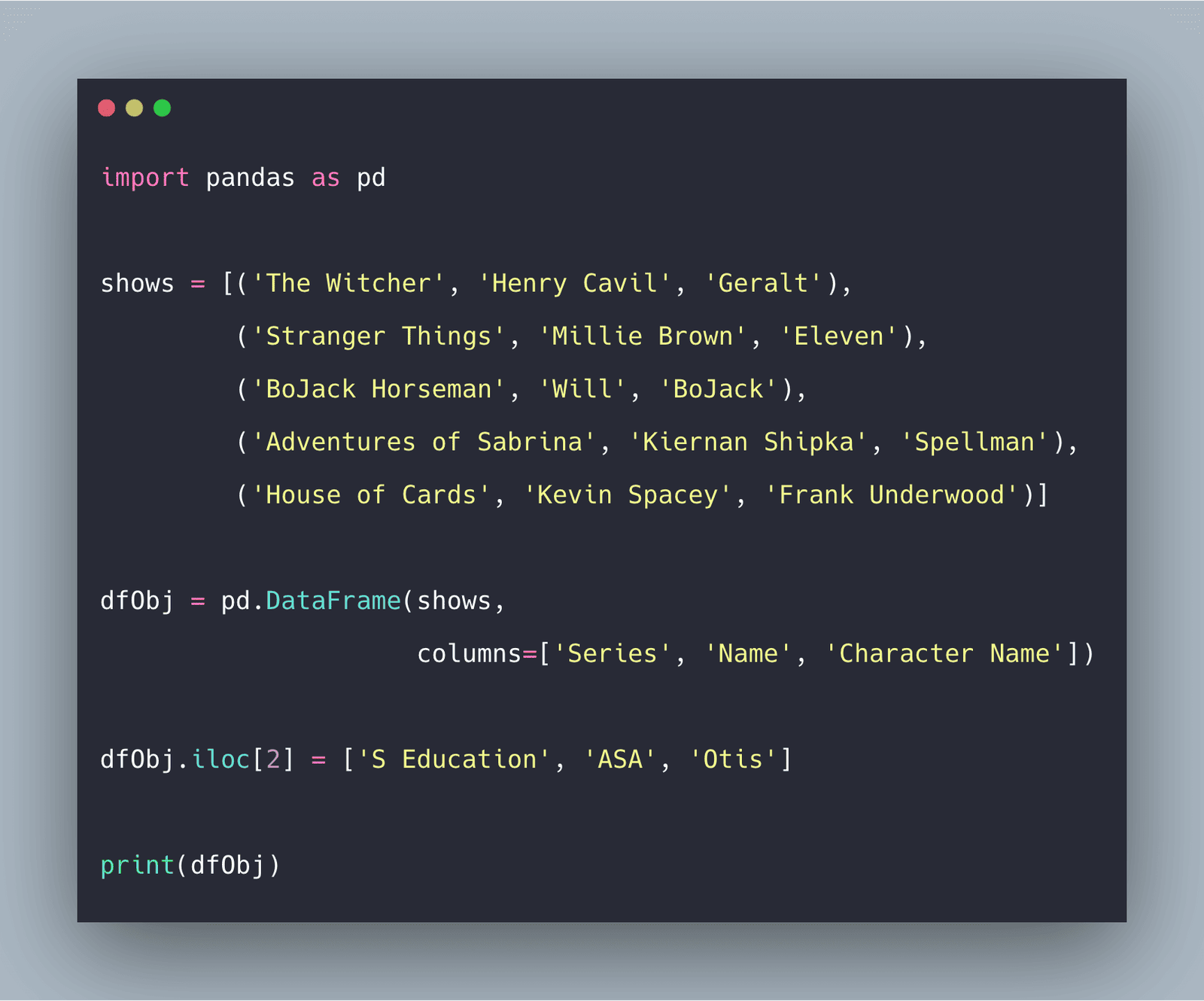# Python Pandas: How To Add Rows In DataFramePython Pandas dataframe append() is an inbuilt function that is used to add rows in the dataframe. The loc[] and iloc[] is also way to add or modify rows.

Python Pandas dataframe append() is an inbuilt function that is used to append rows of other dataframe to the end of the given dataframe, returning a new dataframe object. Columns not in the original dataframes are added as new columns, and the new cells are populated with NaN value.

### How To Add Rows In DataFrame

Python Pandas  DataFrame is a two-dimensional size-mutable, potentially composite tabular data structure with labeled axes (rows and columns). The DataFrame can contain the following types of data.

1. The Pandas Series: One-dimensional labeled array capable of holding any data type with axis labels or index. An example of the Series object is one column from the DataFrame.
2. NumPy ndarray, which can be the record or structure.
3. The two-dimensional ndarray using **NumPy.**
4. Dictionaries of one-dimensional ndarray’s,  lists,  dictionaries, or  Series.

There are mainly three methods we can use to add rows in  DataFrame.

1. Dataframe.append()
2. loc[ ]
3. iloc[ ]

## Python Pandas Objects - Pandas Series and Pandas Dataframe

In this post, we will learn about pandas’ data structures/objects. Pandas provide two type of data structures:- ### Pandas Series Pandas Series is a one dimensional indexed data, which can hold datatypes like integer, string, boolean, float...

## Pandas in Python

Pandas is a fast, powerful, flexible and easy to use open source data analysis and manipulation tool, built on top of the Python programming language.

## Python for Beginners | Numpy and Pandas in Python | Python Basics

We will be working with pandas and NumPy libraries. These libraries are essential for performing exploratory data analysis. We will see how can we create different arrays and manipulate them. With pandas, we will work with both Series and data frames.

## Python Pandas Tutorial (Part 6): Add/Remove Columns From DataFrames

In this video, we will be learning how to add and remove our rows and columns. This video is sponsored by Brilliant. Go to https://brilliant.org/cms to sign ...

## Pandas DataFrame to_numpy() | DataFrame to Numpy Array

Pandas Dataframe.to_numpy() is an inbuilt method that is used to convert a DataFrame to a numpy array. The DataFrame is a two-dimensional data structure.